# JS语言理解15 连等赋值

## 题目

var a = {n: 1};

var b = a;

a.x = a = {n: 2};

console.log(a.x);
console.log(b.x);


## 关键原则

（1）赋值表达式

A = B


1. 计算表达式A，得到一个引用refA
2. 计算表达式B，得到一个值valueB
3. value赋给refA指向的名称绑定
4. 返回valueB

（2）赋值运算右结合，赋值时从右至左永远只取等号右边的表达式结果赋值到等号左侧

let a = b = 1;

console.log(a);
console.log(window.a);

console.log(b)
consoel.log(window.b);


Exp1 = Exp2 = Exp3 = Exp4


Exp1 = (Exp2 = (Exp3 = Exp4))


1. 计算Exp1，得到Ref1
2. 计算Exp2，得到Ref2
3. 计算Exp3，得到Ref3
4. 计算Exp4，得到value4

Ref1 = (Ref2 = (Ref3 = Value4))


1. Value4赋值给Exp3
2. Value4赋值给Exp2
3. Value4赋值给Exp1
4. 返回表达式最终结果value4

（3）声明提前

let a = {};
a.x = 1;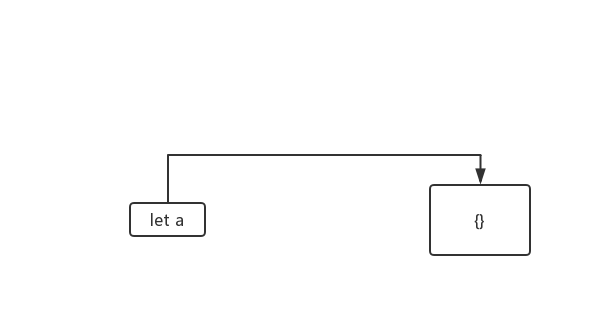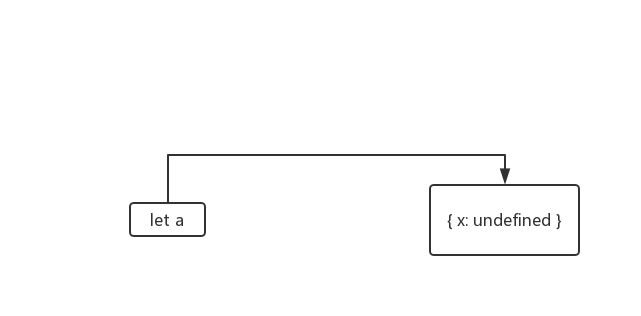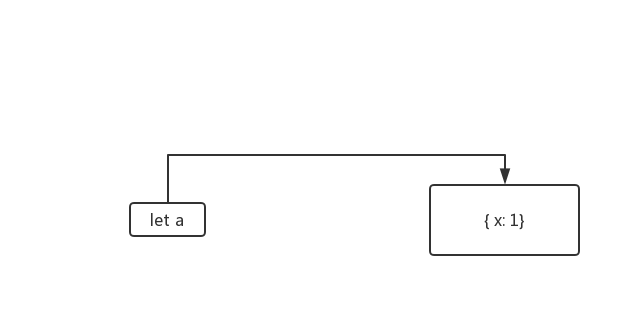## 题目分析

let a = (b = 1);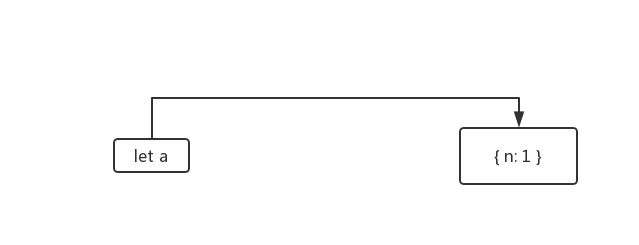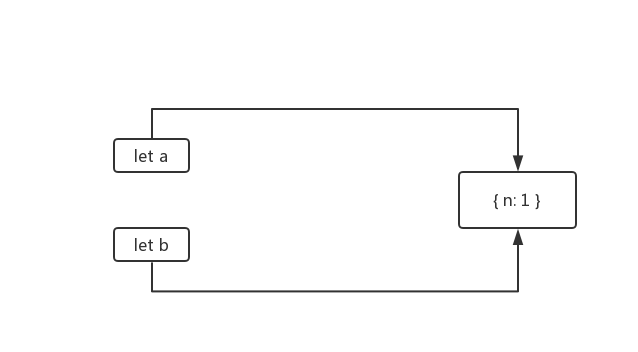a.x = a = {n: 2};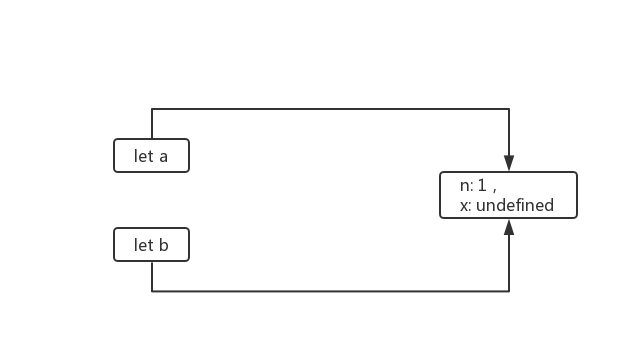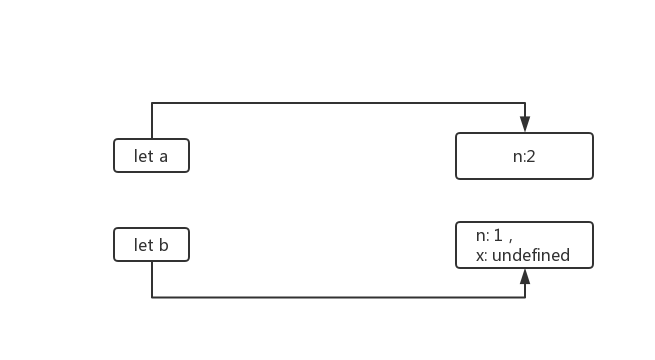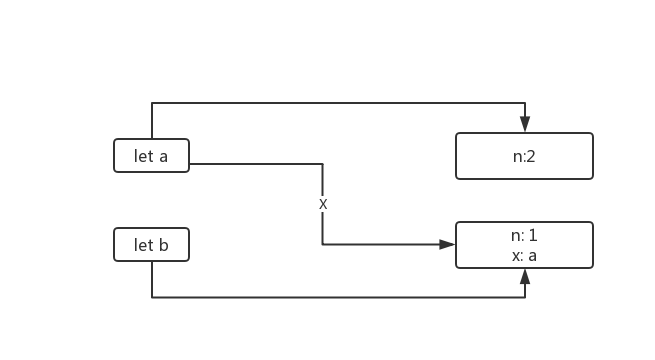## 参考©️2019 CSDN 皮肤主题: 编程工作室 设计师: CSDN官方博客